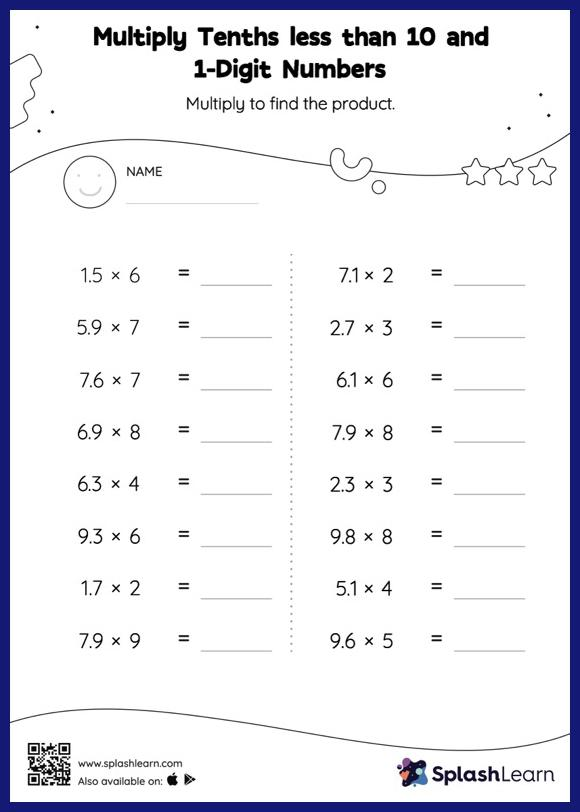# Multiply Tenths less than 10 and 1-Digit Numbers: Horizontal Multiplication Worksheet

Home > Multiply Tenths less than 10 and 1-Digit Numbers: Horizontal MultiplicationThis worksheet consists of a set of questions on multiplication where students are required to multiply tenths less than 10 and 1-digit numbers. Students may omit the decimal point when multiplying a decimal by a one-digit number and then add it when writing the answer. This multiply tenths less than 10 and 1-digit numbers worksheet gives learners many opportunities to practice this concept.